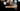# Leetcode | Solution of Find Numbers with Even Number of Digits in JavaScript

April 7th, 2020
|

In this post, we will solve Find Numbers with Even Number of Digits from leetcode and compute it's time and space complexities. Let's begin.

# Problem Statement

The question can be found at leetcode Find Numbers with Even Number of Digits problem.

The problem states that we are given an array of numbers and we need to return the count of numbers that have an even number of digits.

# Solution

The approach to this problem is very straight forward. We loop over the array and for each number, we count the number of digits in the number and check if it is even.

To get all the digits in the number we can take the modulus of the number by `10` and that would give us the last digit. Next, we divide the number by `10` and it gives the new number without the last digit and we repeat the process until the number is `0`.

We have discussed the approach, I urge you to go ahead on leetcode and give it another try.If you are here, it means something went wrong in implementation or you are just too lazy. In any case, let's see a simple implementation of the above logic.
``````var findNumbers = function (nums) {
let count = 0;
for (let num of nums) {
let digit = 0;

while (num > 0) {
digit++;
num = parseInt(num / 10);
}

if (digit % 2 === 0) count++;
}

return count;
};``````

Let's look at the solution. First, we declare a variable count and assign it the value `0` to store the result i.e. the count of numbers with even digits. Next, we loop over the nums array and process each number. In the while loop, we count the number of digits in each number. Next, we check if the number of digits is even and increase the count. At last, we return the count. One thing to note is , we didn't use our modulus here because we didn't really need our last digit.

Here are the stats on submission

``````Status: Accepted
Runtime: 68ms
Memory: 36.5MB``````

## Time and space complexity

### Time complexity

We are looping over the nums array once and have a nested while loop inside the outer loop. On the first look, the complexity looks quadratic. If we look closely, each number is the array is in the range `1 to 100000` i.e. the while loop will run maximum `6` times for the largest number in the array. In the worst case the complexity would be O(6*n), here `6` is a constant, so time complexity would be O(n).

### Space complexity

We are using extra space to store a couple of variables. So space complexity would be constant, O(1).

# Summary

So, we solved the Find Numbers with Even Number of Digits and calculated the time and space complexities.

I hope you enjoyed solving this question. This is it for this one, complete source code for this post can be found on my Github Repo. Will see you in the next one.